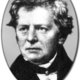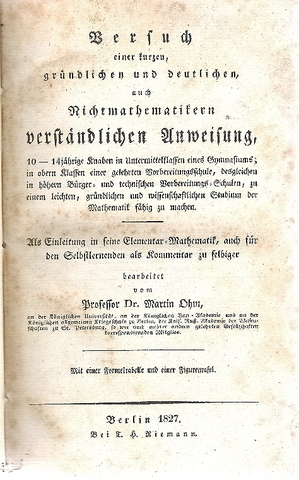By s510296
• # Georg is bornGeorge Ohm was born in Erlangen, Germany
• Period: to

• # Jesuit Gymnasium of CologneOhm is offered a position of a teacher in mathematics and physics at the Jesuit Gymnasium of Cologne
• # Contribution to Science of ElectricityGeorg Simon Ohm, more commonly known as Georg Ohm, was a German physicist, best known for his “Ohm’s Law”, which implies that the current flow through a conductor is directly proportional to the potential difference (voltage) and inversely proportional to the resistance. The physical unit of electrical resistance, the Ohm, also was named after him.
• # Royal SocietyOhm received the Copley Medal award by the Royal Society.
The unit ohm is named after Georg Ohm.
A law relating the voltage difference between two points, the electric current flowing between them, and the resistance of the path of the current. Mathematically, the law states that V = IR, where V is the voltage difference, I is the current in amperes, and R is the resistance in ohms. For a given voltage, higher resistance entails lower current flow.
• # Georg diesGeorg Ohm died in Munich, Germany